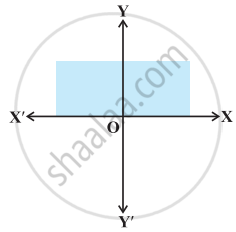# Graph of y ≤ 0 is - Mathematics

MCQ
True or False

Graph of y ≤ 0 is• True

• False

#### Solution

This statement is False.

Explanation:

The given graph represents y ≥ 0

Concept: Graphical Solution of Linear Inequalities in Two Variables
Is there an error in this question or solution?

#### APPEARS IN

NCERT Mathematics Exemplar Class 11
Chapter 6 Linear Inequalities
Exercise | Q 31.(xii) | Page 112

Share